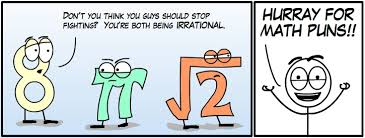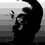# Mathematics PunsWhen the statistics professor and the math professor wrote a cookbook together, they called it 'Pi A La Mode.'

Deaf mathematicians communicate through sin language.

A summer is a mathematician

The marine biology student took a math course called algae-bra.

Some mathematicians are reluctant to cosine a loan.

I was kicked out of math class for one too many infractions.

A mathematician that couldn't stop adding up recently went incremental.

I used to hate maths but then I realized decimals have a point.

I didn't understand the math, so the teacher summed it up for me.

What do organic mathematicians throw into their fireplaces? Natural Logs.

In high school I recall having a beautiful but difficult math teacher. She was easy on the eyes and hard on the pupils!

Mathematicians are sum worshippers.

I strongly dislike the subject of math, however I am partial to fractions.

You know what happens after you miss math class? It starts adding up.

I've failed the mathematics test so many times I lost count.

The mathematician worked at home because he only functioned in his domain.

The math teacher was a good dancer - he had algorithm.

I just finished reading Newton's Principia Mathematica, and found much of it to be rather derivative.

I'm bad at math, so the equation 2n+2n is 4n to me.

The best place for a mathematician is behind a counter.

The mathematician did not practice safe six and ended up with a binarial disease.

Math class is full of drama. There are so many problems to work out.

The arrogant math teacher finally ate a slice of humble pi.

The top maths student's blood type was A+.

The inept mathematician couldn't count on his friends.

The math teacher was hungry, but all she had to eat was a piece of pi.

The first order of priority in hiring math majors is get them to sine on the dotted line

I met a math professor who has 12 children - she really knows how to multiply.

A rubber band pistol was confiscated from algebra class because it was a weapon of math disruption.

We'll never run out of math teachers because they always multiply.

Old math professors never die, they just reduce their functions.

He became a math teacher due to some prime factors.

A lawyer was defending a math teacher. He had to sum up.

On the shelf there are ten math books, five geography books, and the rest is history.

The math professor went crazy with the blackboard. He did a number on it.

Old math teachers never die, they just become irrational.

The math professor liked even numbers, but only the odd one.

Two mathematicians arguing about even numbers were at odds.

With negative numbers, some math students become nonplussed.

A mountain climbing math teacher found an adder at the sum-mit.

Old math profs never die, they just can't differentiate.

He wears glasses during math because it improves division.

The math teacher was an exponent of his own powers.

His qualifications as a math teacher didn't add up.

Old math profs never die -- they just use strange expressions.

Mathematics teachers call retirement 'the aftermath'.

Young women who are mathematics professors closely watch their figures.

A math professor in an unheated room is cold and calculating.

Math teachers have lots of problems.

Some mathematicians are on the negative side while others are quite positive.

A mathematician who was also a horticulturist was interested in prime roots square roots and trees in general.Note by Lew Sterling Jr
6 years, 8 months ago

This discussion board is a place to discuss our Daily Challenges and the math and science related to those challenges. Explanations are more than just a solution — they should explain the steps and thinking strategies that you used to obtain the solution. Comments should further the discussion of math and science.

When posting on Brilliant:

• Use the emojis to react to an explanation, whether you're congratulating a job well done , or just really confused .
• Ask specific questions about the challenge or the steps in somebody's explanation. Well-posed questions can add a lot to the discussion, but posting "I don't understand!" doesn't help anyone.
• Try to contribute something new to the discussion, whether it is an extension, generalization or other idea related to the challenge.

MarkdownAppears as
*italics* or _italics_ italics
**bold** or __bold__ bold
- bulleted- list
• bulleted
• list
1. numbered2. list
1. numbered
2. list
Note: you must add a full line of space before and after lists for them to show up correctly
paragraph 1paragraph 2

paragraph 1

paragraph 2

[example link](https://brilliant.org)example link
> This is a quote
This is a quote
    # I indented these lines
# 4 spaces, and now they show
# up as a code block.

print "hello world"
# I indented these lines
# 4 spaces, and now they show
# up as a code block.

print "hello world"
MathAppears as
Remember to wrap math in $$ ... $$ or $ ... $ to ensure proper formatting.
2 \times 3 $2 \times 3$
2^{34} $2^{34}$
a_{i-1} $a_{i-1}$
\frac{2}{3} $\frac{2}{3}$
\sqrt{2} $\sqrt{2}$
\sum_{i=1}^3 $\sum_{i=1}^3$
\sin \theta $\sin \theta$
\boxed{123} $\boxed{123}$

Sort by:

Nice!From where did you get these?@Llewellyn Sterling

- 6 years, 8 months ago